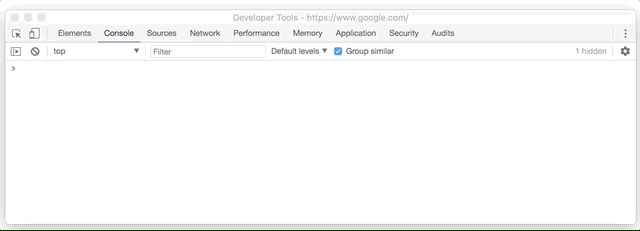Lesson 3

# JavaScript Programming Fundamentals: Operators & If Else

If you like what I'm doing here, or want to join the discussion, you can become a patron at http://patreon.com/coreyhowell. Patrons make it possible for me to keep doing what I love, spreading my knowledge!## Operators

We’re all familiar with some operators. You know the following symbols + - ÷, they’re math operators that indicate the kind of math you want to do with given variables. Programmers rely on many operators, and many of them don’t involve math at all, but instead they involve comparisons, logic. As usual, code speaks louder than words. Lets crush some out!

### Assignment Operator

Type Description
= we’ve seen this one already, assign a value to a variable or property.

### Comparison Operators

Recall booleans from the previous lesson. A boolean is true or false, sometimes they are also referred to as logical values. Comparison operators are binary operators that compare two operands and return the logical, or boolean, value of the expression… Okay, I know that was jargon. Jargon is good for you, call people unevaluated expressions under your breath.

Also, don’t be confused by the term binary operator, it just means it evaluates two operands whereas a unary operator only has one operand, we’ll see unary operators later. I’m going to write a bit of code and label the jargon, ready?

Copy and paste the above code into the console in Google Chrome and press Enter/Return. Then type myScoreWins into the console and press Enter/Return. You should see this:Our expression says myScore is greater than highScore evaluates to true. So, myScoreWins has a value of true.
If we were to flip the position of the values in our expression, we’d see this:
highScore is greater than myScore evaluates to false. So, myScoreWins has a value of false.

Type Description example
=== Comparing two values are equal. 1 === 2 is false
!== Comparying two values are not equal. 1 !== 2 is true
> Value on left is greater than value on right. 1 > 2 is false
>= Value on left is greater than or equal to value on right. 1 >= 2 is false
< Value on left is less than value on right. 1 < 2 is true
<= Value on left is less than or equal to value on right. 1 <= 2 is true

### Arithmetic Operators

Sometimes you gotta do math. Its a bummer but life just be like that. Some quick maths:

Type Description example
+ Add two operands together. 10 + 2 is 12
- Subtract the second operand from the first. 10 - 2 is 8
* Multiply two operands together. 10 * 2 is 20
/ Divide the operand on the left by the operand on the right. 10 / 2 is 5
% Modulo. Divide the operand on the left by the operand on the right return the remainder. 10 % 3 is 1
++ Add 1 to a value. 10++ is 11
-- Subtract 1 from a value. 10-- is 9

A note on the ++ and – operators:

Congratulations, now you know math.

### Logical Operators

Logical operators take two values (if binary) or one value (if unary) and return a boolean. They’re useful for performing logical determinations within code, such as if you need to know if someone is old enough and logged in to see a video game ad, for example.

allowEntry is true above because loggedIn is true AND age is higher than 18. && being our operator.

Type Description example
&& Left AND right operand must be true, to return true. true && false is false
|| Left OR right operand must be true, to return true. true || false is true
! Unary operator called NOT. Returns false if its operand is true, otherwise its true. !true is false

## if else

Logical determinations are what programming is all about. Being able to do something IF a condition is met, or something ELSE if it is not, is the entire point of the IF ELSE statement.

Lets talk about something that i’ve done here, you can see this bit of code:

`(coffeeCount * coffeeCost)`

Notice that it is wrapped in its own parethesis. This is for grouping together sets of logic. Its not necessary here but it sure makes it easy to read. You can easily tell that I first want to evaluate the total of coffeeCount and coffeeCost and then determine if it is less than or equal to myMoney.

Again, feel free to open the console right on this page, or put this code in your main.js. Remember to refresh the page each time you want to run the code.

## Conclusion

Let’s make tacos.

Feel free to replace the code in your main.js file with these code snippets and play around with the values, or just paste them into a console (refresh the page/console to try new values).

If you have any questions or comments, click the "Go To Discussion" button below!